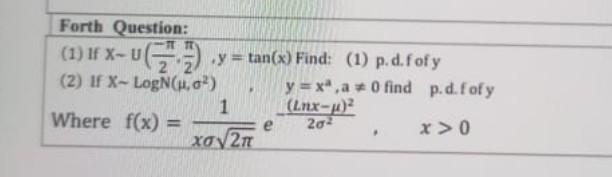# (Solved):   Forth Question: (1) If $$x-U\left(\frac{-\pi}{2}, \frac{\pi}{2}\right), y=\tan (x)$$ Fin ...Forth Question: (1) If $$x-U\left(\frac{-\pi}{2}, \frac{\pi}{2}\right), y=\tan (x)$$ Find: (1) p.d.f of $$y$$ (2) If $$x-\operatorname{LogN}\left(\mu, \sigma^{2}\right), y=x^{a}, a \neq 0$$ find p.d.f of $$y$$ Where $$f(x)=\frac{1}{x \sigma \sqrt{2 \pi}} e^{-\frac{(\ln x-\mu)^{2}}{2 \sigma^{2}}}, \quad x>0$$

We have an Answer from Expert